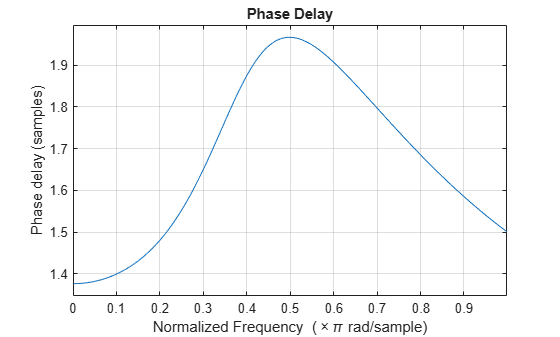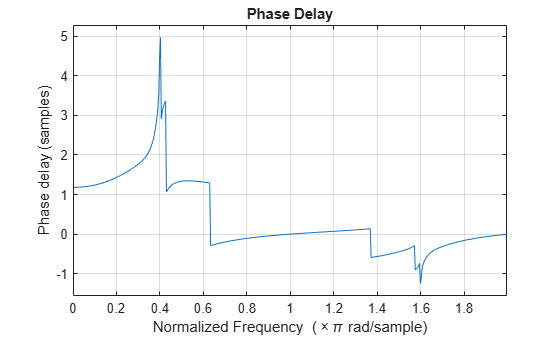# phasedelay

Phase delay of digital filter

## Syntax

``[phi,w] = phasedelay(b,a,n)``
``[phi,w] = phasedelay(sos,n)``
``[phi,w] = phasedelay(d,n)``
``[phi,w] = phasedelay(___,n,'whole')``
``[phi,f] = phasedelay(___,n,fs)``
``[phi,f] = phasedelay(___,n,'whole',fs)``
``phi = phasedelay(___,w)``
``phi = phasedelay(___,f,fs)``
``[phi,w,s] = phasedelay(___)``
``[phi,f,s] = phasedelay(___)``
``phasedelay(___)``

## Description

````[phi,w] = phasedelay(b,a,n)` returns the `n`-point phase delay response vector `phi` and the corresponding `n`-point angular frequency vector `w` for the digital filter with transfer function coefficients stored in `b` and `a`.```

example

````[phi,w] = phasedelay(sos,n)` returns the `n`-point phase delay response corresponding to the second-order sections `sos`.```
````[phi,w] = phasedelay(d,n)` returns the `n`-point phase delay response of the digital filter `d`.```

example

````[phi,w] = phasedelay(___,n,'whole')` returns the phase delay response at `n` equally spaced points around the whole unit circle.```
````[phi,f] = phasedelay(___,n,fs)` returns the phase delay response and the corresponding `n`-point frequency vector `f` for a digital filter designed to filter signals sampled at a rate `fs`.```
````[phi,f] = phasedelay(___,n,'whole',fs)` returns the frequency vector `f` at `n` points ranging between `0` and `fs`.```
````phi = phasedelay(___,w)` returns the phase delay response evaluated at the angular frequencies specified in `w`.```
````phi = phasedelay(___,f,fs)` returns the phase delay response evaluated at the frequencies specified in `f`.```
````[phi,w,s] = phasedelay(___)` returns plotting information, where `s` is a structure with fields that you can change to display different frequency response plots.```
````[phi,f,s] = phasedelay(___)` returns plotting information, where `s` is a structure with fields that you can change to display different frequency response plots.```

example

````phasedelay(___)` plots the phase delay response versus frequency.```

## Examples

collapse all

Use constrained least squares to design a lowpass FIR filter of order 54 and normalized cutoff frequency 0.3. Specify the passband ripple and stopband attenuation as 0.02 and 0.08, respectively, expressed in linear units. Compute and plot the phase delay response of the filter.

```Ap = 0.02; As = 0.008; b = fircls1(54,0.3,Ap,As); phasedelay(b)```Repeat the example using `designfilt`. Keep in mind that this function expresses the ripples in decibels.

```Apd = 40*log10((1+Ap)/(1-Ap)); Asd = -20*log10(As); d = designfilt('lowpassfir','FilterOrder',54,'CutoffFrequency',0.3, ... 'PassbandRipple',Apd,'StopbandAttenuation',Asd); phasedelay(d)```Design a third-order lowpass Butterworth filter with a cutoff frequency of 200 Hz. The sample rate is 1000 Hz.

```fc = 200; fs = 1000; [z,p,k] = butter(3,fc/(fs/2),'low');```

Use the `zp2sos` function to convert the zeros, poles, and gain to second-order sections. Compute the phase delay response of the filter and set the number of evaluation points to `1024`. Display the result.

```sos = zp2sos(z,p,k); phasedelay(sos,1024)```Design an elliptic filter of order 10 and normalized passband frequency 0.4. Specify a passband ripple of 0.5 dB and a stopband attenuation of 20 dB. Display the phase delay response of the filter over the complete unit circle.

```[b,a] = ellip(10,0.5,20,0.4); phasedelay(b,a,512,'whole')```Repeat the example using `designfilt`.

```d = designfilt('lowpassiir','DesignMethod','ellip','FilterOrder',10, ... 'PassbandFrequency',0.4, ... 'PassbandRipple',0.5,'StopbandAttenuation',20); phasedelay(d,512,'whole')```## Input Arguments

collapse all

Transfer function coefficients, specified as vectors.

Data Types: `single` | `double`

Number of evaluation points, specified as a positive integer. Set `n` to a value greater than the filter order.

Data Types: `single` | `double`

Second-order section coefficients, specified as a matrix. `sos` is a K-by-6 matrix, where K is the number of sections and must be greater than or equal to 2. If the number of sections is less than 2, the function considers the input to be a numerator vector, `b`. Each row of `sos` corresponds to the coefficients of a second-order (biquad) filter. The ith row of the `sos` matrix corresponds to ```[bi(1) bi(2) bi(3) ai(1) ai(2) ai(3)]```.

Data Types: `single` | `double`

Digital filter, specified as a `digitalFilter` object. To generate `d` based on frequency-response specifications, use the `designfilt` function.

Angular frequencies at which the function evaluates the phase delay response, specified as a vector and expressed in rad/sample. The frequencies are normally between 0 and π. `w` must contain at least two elements.

Sample rate, specified as a real-valued scalar and expressed in hertz.

Data Types: `double`

Frequencies at which the function evaluates the phase delay response, specified as a vector and expressed in hertz. `f` must contain at least two elements.

## Output Arguments

collapse all

Phase delay response, returned as a vector of length `n`. The phase delay response is evaluated at `n` equally spaced points around the upper half of the unit circle.

Note

If the input to `phasedelay` is single precision, the function calculates the phase delay response using single-precision arithmetic. The output `phi` is single precision.

Angular frequencies in rad/sample, returned as a vector. If you specify `n`, `w` has length `n`. If you do not specify `n` or you specify `n` as an empty vector, then `w` has length `512`.

Frequencies in hertz, returned as a vector. If you specify `n`, `f` has length `n`. If you do not specify `n` or you specify `n` as an empty vector, then `f` has length `512`.

Plotting information, returned as a structure. You can modify the fields in `s` to display different frequency response plots.

## Algorithms

The phase delay response of a filter corresponds to the time delay that each frequency component experiences as the input signal passes through the system. The `phasedelay` function returns the phase delay response and the frequency vector of the filter

`$H\left({e}^{j\omega }\right)=\frac{B\left({e}^{j\omega }\right)}{A\left({e}^{j\omega }\right)}=\frac{\text{b}\left(1\right)+\text{b}\left(2\right){e}^{-j\omega }+\dots +\text{b}\left(\text{m}+1\right){e}^{-jm\omega }}{\text{a}\left(1\right)+\text{a}\left(2\right){e}^{-j\omega }+\dots +\text{a}\left(\text{n}+1\right){e}^{-jn\omega }}$`

given numerator and denominator coefficients in inputs `b` and `a`.

## Version History

Introduced before R2006a